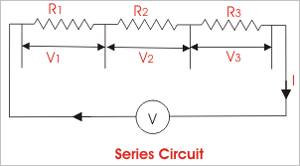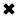# Resistance in Series ExplainedResistance in Series - Parallel

When you place R-I-S, their ohmic values add up arithmetically to get to the total (or net) resistance.

We can connect series of resistors, (equal to the sum of the individual resistances of a parallel circuit) all having identical ohmic values, in parallel sets of series networks, or in series sets of parallel networks. When we do either of these things, we get a series-parallel network that can greatly increase the total power handling capacity of the network over the power-handling capacity of a single parallel resistor.Fig. 4-14. Three resistors in series.

Sometimes, the total single equivalent resistance of the combination circuit in a series parallel network equals the value of any one of the resistors. This always happens if the parallel branches or parallel combinations of the connection components are all identical, and are arranged in a network called an n-by-n (or n x n) matrix. That means when n is a whole number, we have n series sets of n resistors connected in parallel, or else we have n parallel sets of n resistors connected in series resistance of the circuit. These two arrangements yield the same practical result for electrical circuits.

A combination of series parallel combinations array of n by n resistors, all having identical ohmic values and identical power ratings, will have n2 times the power-handling capability of any resistor by itself. For example, a 3 x 3 series-parallel matrix of 2 W resistors can handle up to 32 x 2 = 9 x 2 = 18 W. If we have a 10 x 10 array of 1/2 W resistors, then it can dissipate up to 102 x 1/2 = 50 W. We multiply the power-handling capacity of each individual resistor by the total number of resistors in the matrix.

The above-described scheme works if, but only if, all of the resistors have identical ohmic values according to ohms law and identical power-dissipation ratings in terms of total voltage drops when the sum of the voltage drops across each resistor. If the resistors have values that differ even a little bit from one another, one of the components will likely draw more current than it can withstand, so it will burn out, no matter the voltage source. Then the current distribution in the network will change further, increasing the likelihood that a second resistor will fail, and maybe more.

If you need a resistor that can handle 50 W and a certain series-parallel connection of the network will handle 75 W, that's fine. But you should not "push your luck" and expect to get away with using a network that will handle only 48 W in the same application. You should allow some extra tolerance, say 10 per cent over the minimum rating. If you expect the network to dissipate 50W, you should build it to handle 55 W or a bit more. You don't have to use "overkill," however. You'll waste resources if you cobble together a network that can handle 500W when you only expect it to cope with 50W—unless that's the only convenient combination you can make with available resistors.

##### Basic Electricity Handbook, Vol. 1• FULLY ILLUSTRATED
• GREAT FOR SCHOOL PROJECTS
• GREAT PRICE: \$5.99
• ...

This 100+ page e-book is a great guide for those who have a basic interest in the field of electricity. This well-illustrated e-book, coupled with some basic knowledge of electricity, will give you a broad theoretical background in this fundamental subject.

CONTENTS
• FUNDAMENTALS OF ELECTRICITY
• HOW DOES ELECTRICITY WORK?
• HOW IS ELECTRICITY GENERATED?
• WHERE DOES ELECTRICITY COME FROM?
• BASIC HOME WIRING
• ELECTRICITY FOR STUDENTS
• ENERGY SAVING TIPS

##### TRAINING EF COURSES

Content Community Connection
Top